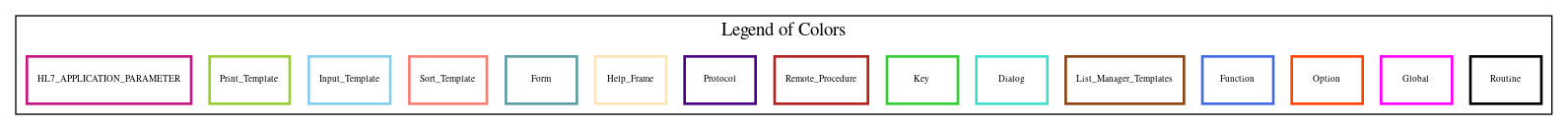Home   Package List   Routine Alphabetical List   Global Alphabetical List   FileMan Files List   FileMan Sub-Files List   Package Component Lists   Package-Namespace Mapping
Routine: PRCHDP9

# Routine: PRCHDP9

## Information

PRCHDP9 ;WISC/DJM-PRINT AMENDMENT,ROUTINE #5 ;12/12/95 9:22 AM

## Source Information

Source file <PRCHDP9.m>

## Call Graph### Call Graph Total: 2

Package Total Call Graph
IFCAP 2 (DATA,LCNT,LCNT1,LINE)^PRCHDP9  LINE^PRCHPAM5

## Caller Graph

### Legends:#### Package Component Superscript legend

 action A extended action Ea event driver Ed subscriber Su protocol O limited protocol LP run routine RR broker B edit E server Se print P screenman SM inquire I### Caller Graph Total: 5

Package Total Caller Graph
IFCAP 5 PRCHDP5  PRCHDP6  PRCHDP7  PRCHDP8  PRCHDP9

## Entry Points

V ;;5.1;IFCAP;;Oct 20, 2000
E34 ;AUTHORITY Edit PRINT
CHK(DATA,DT2) ;
E35 ;F.O.B. Point PRINT
E37 ;ITEM DISCOUNT Delete PRINT
LCNT(LCNT) ;FETCH THE CURRENT LINE COUNT FROM "W" ARRAY
LINE(LCNT,LINES) ;ADDS A NUMBER OF BLANK "LINES" INTO "W" ARRAY
DATA(LCNT,DATA) ;PLACES THE AMENDMENT LINE OF TEXT INTO THE "W" ARRAY
LCNT1(LCNT) ;PUT BACK LCNT INTO "W" ARRAY

## External References

Name Field # of Occurrence
DATA^PRCHDP9 E34+7, E34+8, E34+9, E35+7, E36+23, E36+24, E36+26, E36+27, E36+29, E36+31
E37+10, E37+11
LCNT^PRCHDP9 E34+2, E35+3, E36+2, E37+2
LCNT1^PRCHDP9 E34+13, E35+8, E36+33, E37+11
LINE^PRCHDP9 E34+6, E36+20, E37+9
LINE^PRCHPAM5 E35+6

## Global Variables Directly Accessed

Name Line Occurrences  (* Changed,  ! Killed)
^PRC(442 - [#442] E34+3, E34+4, E34+5, E35+4, E35+5, E36+3, E36+4, E36+5, E36+6, E36+12
E36+17, E36+18, E36+19, E37+3, E37+4, E37+5, E37+7, E37+8
^PRCD(442.2 - [#442.2] E34+8, E34+12
^TMP(\$J LCNT+1, LINE+2*, DATA+1*, LCNT1+1*

## Label References

Name Line Occurrences
CHK E34+12
DATA E34+11, CHK+2

## Local Variables

### Legend:

 >> Not killed explicitly * Changed ! Killed ~ Newed

Name Field # of Occurrence
>> AMEND E34+3, E35+4, E36+3, E36+4, E37+3, E37+4
CHANGE E34+2*, E34+3*, E34+4, E35+3*, E35+4*, E35+5, E36+1~, E36+4*, E36+5, E36+6
E37+4*, E37+5
CHANGES E34+4*, E36+1~, E36+5*, E36+11, E37+5*, E37+6
DATA E34+7*, E34+8*, E34+9*, E34+10*, E34+11*, E34+12, CHK~, CHK+1*, CHK+2*, E35+7*
E36+1~, E36+23*, E36+24*, E36+26*, E36+27*, E36+29*, E36+31*, E37+10*, E37+11*, DATA~
, DATA+1
DIS E36+1~, E37+8*, E37+11
DIS1 E36+1~
DIS2 E36+1~, E36+7!, E36+8*, E36+21*, E36+26, E36+31
DT2 E34+8*, E34+9, E34+10, E34+12*, CHK~, CHK+1, CHK+2
FIELD E36+1~, E36+2*, E36+3*, E36+4, E36+8, E36+9, E36+17, E37+2*, E37+3*, E37+4
FLAGDISC E36+1~, E36+10*, E36+23
I E34+12*, LINE+1~, LINE+2*
ITEMD E36+1~, E36+2!, E36+11*, E36+12, E36+17, E36+19, E37+2!, E37+6*, E37+7, E37+8
ITEMD( E36+11*
ITEMD0 E36+1~, E36+12*, E37+7*
LCNT E34+2, E34+6, E34+7, E34+8, E34+9, E34+11, E34+13, CHK+2, E35+3, E35+6
E35+7, E35+8, E36+1~, E36+2, E36+20, E36+23, E36+24, E36+26, E36+27, E36+29
E36+31, E36+33, E37+2, E37+9, E37+10, E37+11, LCNT~, LCNT+1*, LINE~, LINE+2*
, DATA~, DATA+1*, LCNT1~, LCNT1+1
LINES LINE~, LINE+2
NEW E34+5*, E34+8, E34+12, E35+5*, E35+7, E36+1~, E36+19*
NEWI E36+1~, E36+19*, E36+24, E36+27, E36+29, E36+31
NEWP E36+1~, E36+19*, E36+24, E36+27, E36+29
OLD E34+4*, E34+7, E34+11, E34+12, E35+5*, E35+7, E36+1~, E36+6*, E36+8, E36+9
E36+10, E37+5*, E37+11
PCT E36+1~, E36+17*, E36+18
PCT1 E36+1~, E36+17*
PCT2 E36+1~, E36+7!, E36+9*, E36+18*, E36+21*, E36+26, E36+29
>> PRCHAM E34+3, E34+4, E34+5, E35+4, E35+5, E36+3, E36+4, E36+5, E36+6, E36+17
E36+18, E37+3, E37+4, E37+5
>> PRCHPO E34+3, E34+4, E34+5, E35+4, E35+5, E36+3, E36+4, E36+5, E36+6, E36+12
E36+17, E36+18, E36+19, E37+3, E37+4, E37+5, E37+7, E37+8
U E34+5, E34+8, E34+12, E35+5, E36+11, E36+17, E36+19, E37+6, E37+8